Start typing, then use the up and down arrows to select an option from the list.Jules Bruno
164views
2
6
So we did this one in pure water. But now we're gonna dissolve that same compound, no longer impure water. But in a solution that is 0.20 Mueller and a C l. The beginning is still the same. It's still cocoa. Three solid breaks up into cobalt three plus acquis plus three c l minus a quiz. So that part doesn't change. Now, what's gonna be different is this here is Ionic is well and it breaks up into and a positive and see all negative and notice Here we have a C o negative and here we have a c All negative did the same exact ion. We call this type of problem a common iron effect. And here, we're going to say, is that this mole Arat e is the morality of the n a plus the c l minus. They each have that same polarity. We don't care about DNA because DNA is not found in our equation. But we do care about the C l minus. And since the same ion, if the molar ity of this chloride ion is 20 Mueller than the initial polarity of this clarity on is also 20 Mueller that's a big difference. Cobalt. We don't see any of its common ion. So this is zero. This is a solid. So we ignored. We're making products. So this is gonna be plus X plus three acts because of the coefficient plus x 20 plus three X here. We're gonna say K SP equals just products again because are reacting is a solid Now, here's the thing. Anytime we're dealing with K sp, we're gonna say that any time we have k S P, we can ignore a number in its variable as long as it follows a real number. So here this is an actual number. And because it's an actual number, we could ignore this plus three x. So our calculation just becomes X Times 0. Cute. So again for K S P, As long as there's a number in front of that X variable, we could always ignore that X variable here. This plus X here doesn't have a number in front of it, so we can't ignore. That's why we keep it around. Okay, Now this kid, it's still equals r k s P, which is 2.8 times 10 to the negative 13 which we saw earlier. All we gotta do now is solve for X, so divide both sides by 0.20. Cute. What you should get as your answer is X equals 3.5 times 10 to the negative. 11 Moeller. Now, what you should realize here is common in effect. Look at what are X was before we had the common ion. It was to the negative four. Now, because of the common I, in effect, it becomes to the negative 11. You're gonna say the common I in effect decreases soluble ity. So that's the biggest thing you need to take away from this. Not only how to solve a question like this, but the theory behind it. Kalmunai an effect decreases soluble ity because think about it. This thing gets dropped into a bucket and it wants to break up in tow ions. It's gonna try to break up into a much cobalt three plus and as much CEO minuses as it can. But even before it even starts toe break apart, there's already some C l minus initially, which would mean that this thing does not need to break up as much. That's why it's sigh ability is so much smaller because there was already some chloride ions present before it even began to break apart. And that's the key to this type of question. So remember how to solve a regular K SP question in pure water versus solving a K SP question that's not impure water and has a common iron involved.01:30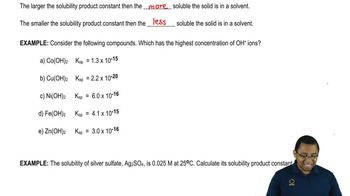01:59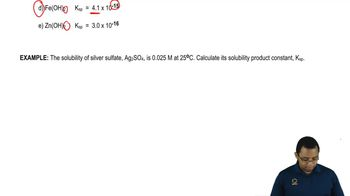05:51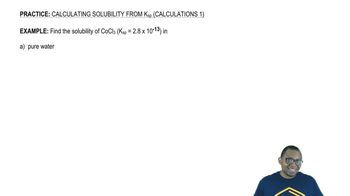05:2104:11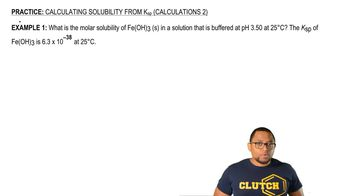04:50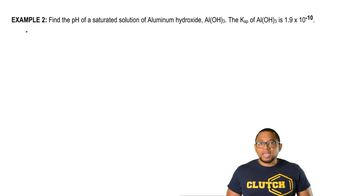05:15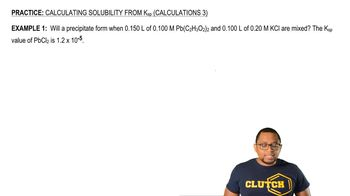07:16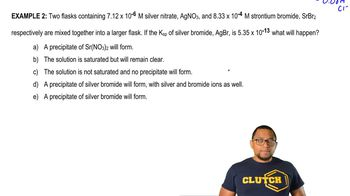06:05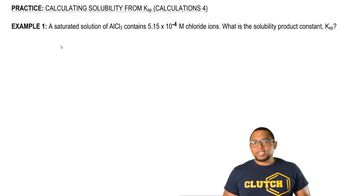03:4704:10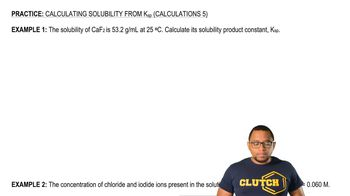03:44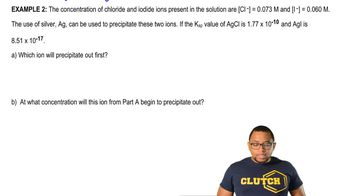03:36